# 6. Binary Trees

This chapter introduces one of the most fundamental structures in computer science: binary trees. The use of the word tree here comes from the fact that, when we draw them, the resulting drawing often resembles the trees we find in a forest. There are lots of ways of defining binary trees. Mathematically, a binary tree is a connected undirected finite graph with no cycles, and no vertex of degree greater than three.

For most computer science applications, binary trees are rooted: A special node,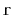, of degree at most two is called the root of the tree. For every node,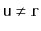, the second node on the path from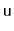tois called the parent of. Each of the other nodes adjacent tois called a child of. Most of the binary trees we are interested in are ordered, so we distinguish between the left child and right child of.

In illustrations, binary trees are usually drawn from the root downward, with the root at the top of the drawing and the left and right children respectively given by left and right positions in the drawing (Figure 6.1). A binary tree with nine nodes is drawn this way in Figure 6.2.a.Figure 6.2: A binary tree with (a) nine real nodes and (b) ten external nodes.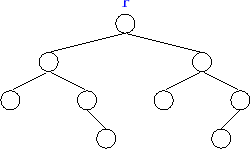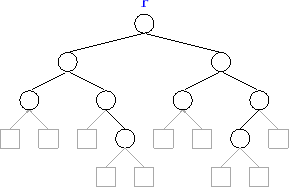(a) (b)

Binary trees are so important that a terminology has developed around them: The depth of a node,, in a binary tree is the length of the path fromto the root of the tree. If a node,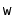, is on the path fromtothenis called an ancestor ofanda descendant of. The subtree of a node,, is the binary tree that is rooted atand contains all of's descendants. The height of a node,, is the length of the longest path fromto one of its descendants. The height of a tree is the height of its root. A node,, is a leaf if it has no children.

We sometimes think of the tree as being augmented with external nodes. Any node that does not have a left child has an external node as its left child and any node that does not have a right child has an external node as its right child (see Figure 6.2.b). It is easy to verify, by induction, that a binary tree having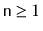real nodes has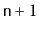external nodes.

Subsections
opendatastructures.org